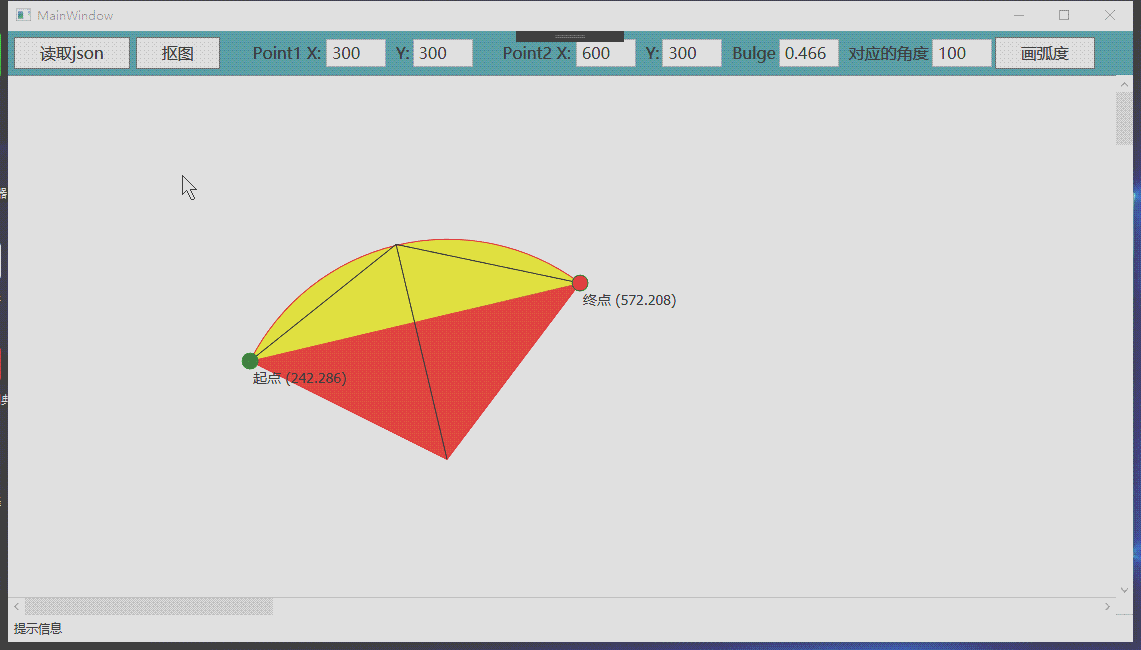1 凸度的概念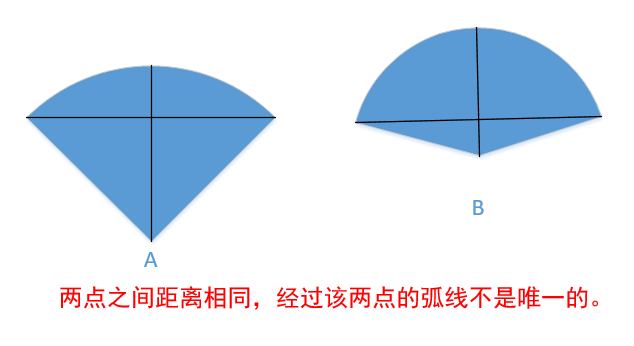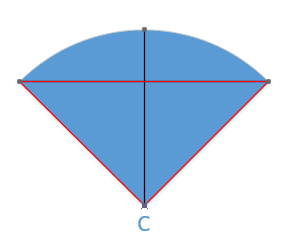2 根据凸度计算及画图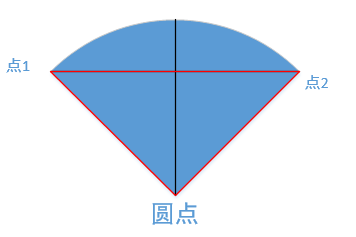WPF绘图上下文类为DrawingContext，这个类有一个绘图函数

public abstract void DrawGeometry(Brush brush, Pen pen, Geometry geometry);

 void AddArc(PathGeometry pathGeometry, System.Windows.Point point1, System.Windows.Point point2,  double bulge, double radius)
{
PathFigure pathFigure = new PathFigure();
pathFigure.StartPoint = point1;//起点

ArcSegment arcSegment = new ArcSegment();
arcSegment.Point = point2; //终点

//半径

//对应的角度
double radian = Math.Atan(bulge) * 4;
double angle = radian * 180 / Math.PI;
arcSegment.RotationAngle =Math.Abs(angle);
//弧度的方向 顺时针还是逆时针
arcSegment.SweepDirection = bulge>0? SweepDirection.Clockwise:SweepDirection.Counterclockwise;
//是否大于180度
arcSegment.IsLargeArc = Math.Abs(bulge) > 1;

}

上述代码根据两点、凸度、圆半径来实现画图。（半径是根据前三个计算出来的，后面会讲该算法）

ArcSegment 还有一个参数就是圆的半径。其实根据两点、’凸度就可推导出半径，不知道为什么ArcSegment 不替我们计算出来。下面讲一下如何计算半径。

3 根据凸度计算半径

  public static double CalBulgeRadius(System.Windows.Point point1, System.Windows.Point point2, double bulge)
{
//计算顶点角度
double cicleAngle = Math.Atan(bulge) * 4;

//两点之间的距离
double pointLen = ImageHelper.CalPointLen(point1, point2);
//根据正玄值反推
double radius = (pointLen / 2) / Math.Sin(cicleAngle / 2);
}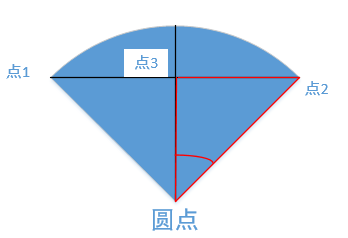看左图，可知：顶点对应的角度的一半的正玄值就是 两点之间的长度除以半径。根据这一点就可以算出半径。

3 计算圆点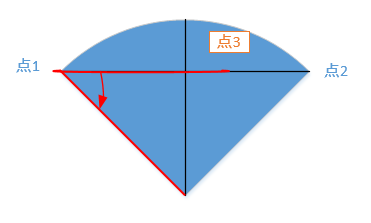//两点之间的距离
double pointLen = ImageHelper.CalPointLen(point1, point2);

//半径与两点之间距离的比值
double lenRate = radius / pointLen;
System.Windows.Point midPoint = ImageHelper.GetMidPoint(point1, point2, lenRate);

internal static System.Windows.Point GetMidPoint(System.Windows.Point pt1, System.Windows.Point pt2, double lenRate)
{
Debug.Assert(lenRate>=0);
if (lenRate == 1)
return pt2;
if (lenRate == 0)
return pt1;

System.Windows.Point result = new System.Windows.Point();
result.X = pt1.X + lenRate * (pt2.X - pt1.X);
result.Y = pt1.Y + lenRate * (pt2.Y - pt1.Y);
return result;
}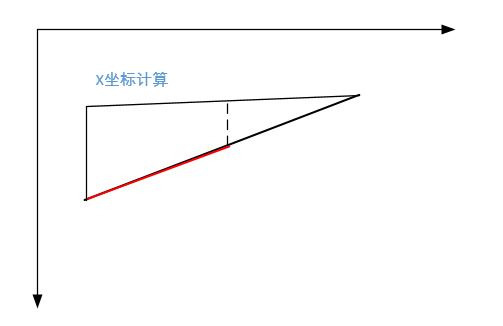public static System.Drawing.Point RotationAt(System.Drawing.Point pointMove, System.Drawing.Point removeAt,
double rotateAngle, bool clockwise)
{
if (rotateAngle == 0)
return pointMove;

lock (matrix)
{
matrix.Reset();
//设置旋转的角度
matrix.Rotate((float)(clockwise ? rotateAngle : -rotateAngle));

//相对于removeAt旋转
System.Drawing.Point pt2 = new System.Drawing.Point(pointMove.X - removeAt.X, pointMove.Y - removeAt.Y);
System.Drawing.Point[] pts = new System.Drawing.Point[] { new System.Drawing.Point(pt2.X, pt2.Y) };
matrix.TransformPoints(pts);

//再变换到圆点位置
System.Drawing.Point result = new System.Drawing.Point(pts.X + removeAt.X, pts.Y + removeAt.Y);
return result;
}
}

后记：在二维坐标上画图，需要有一定的几何基础和空间想象能力。初始接触这类编程，还是有一定难度的。就需要我们补充一些几何知识，同时多思考，总有一天会感觉豁然开朗。

posted @ 2018-12-08 22:37 源之缘 阅读(...) 评论(...) 编辑 收藏